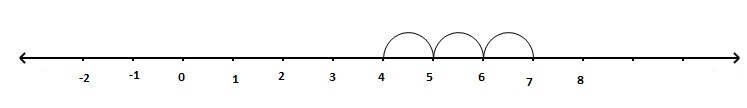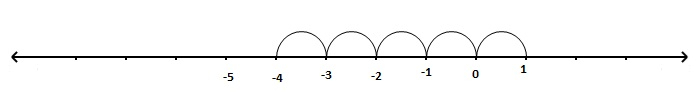# Use the number line and write the number which is: (a) 3 more than 4 (b) 5 less than 1

Given :

The given terms are (a) 3 more than 4  (b) 5 less than 1.

To do :

We have to write the numbers using the number line.

Solution :

(a)3 more than 4

Step 1: First mark 4.

Step 2: Move 3 steps to the right.So, 3 more than 4 is 7.

b) 5 less than 1

Step 1: First mark 1.

Step 2: Move 5 steps to the left.So, 5 less than 1 is $-4$.

Updated on: 10-Oct-2022

148 Views